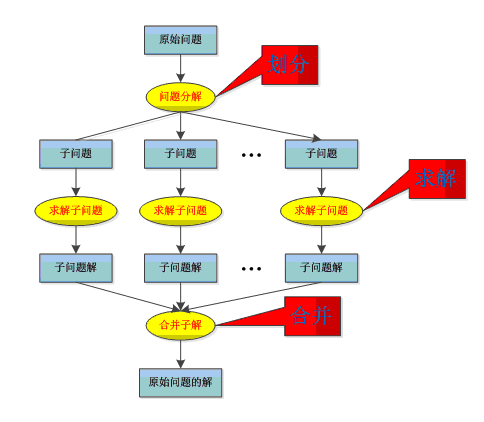1.1分治法基本介绍

1.2分治法通俗解释

1.3分治法严谨定义

1.4分治法的流程

1.5分治法的经典例子

1.6总结

## 3.1栗子

1.将100硬币分成3份，33,33,34。

2.称量1、2份，若天平平衡，则假币必在另外34枚中。若不平衡，假币在轻的那33枚里。

3.将34枚分为11/11/12枚（或将33枚分成11*3）。

4.称量两组11枚的硬币，若平衡，假币在12枚里（或另外的11枚）若不平衡，假币在轻的11里。

5.将11（或12）枚分成3/4/4（或4/4/4），称量4/4，方法同上。

6.将剩下的3（或4）分为1/1/1（或1/1），称量1/1，若平衡，则剩下的一枚是假币，若不平衡，轻的是假币。 若还剩4枚，出现1/1平衡，剩下2枚则称量，显然轻的是假币。

## 3.2栗子分析

1.观察可以看到1-2，3-4，5-6步除了硬币的枚数改变了，其他的步骤完全一样。

2.观察发现这是一个子问题的分解过程，100—33-11-3，将一个大问题分解为了容易解决的小问题。

3.可以发现小问题是相互独立的。每一个33枚硬币和其他的并不相互影响。

## 5.1流程Divide-and-Conquer！

``````Divide-and-Conquer(P)

1. if |P|≤n0

3. 将P分解为较小的子问题 P1 ,P2 ,…,Pk

4. for i←1 to k

5. do yi ← Divide-and-Conquer(Pi) △ 递归解决Pi

6. T ← MERGE(y1,y2,…,yk) △ 合并子问题

7. return(T)

## 5.2使用条件

1.该问题的规模缩小到一定的程度就可以容易地解决

2.该问题可以分解为若干个规模较小的相同问题。

3.利用该问题分解出的子问题的解可以合并为该问题的解；

4.该问题所分解出的各个子问题是相互独立的，即子问题之间不包含公共的子子问题。(非必需）

## 6.1.2思路分析：

1.比较两个数字大小很容易。

2.将n个数字排序和将n/2个数字排序问题相同

3.不存在重叠子问题。

1.分割：将这个序列分割成一个个已经排好序的子序列（即子序列里只有一个数）

2.比较。

3.归并：将一个个有序的子序列合并成排好序的序列。

## 6.1.3coding time

``````//首先来看函数主体，实现了分割的部分
void mergesort(int a[],int n,int left,int right)
{
if(left+1<right)
{
int mid=(left+right)/2;
mergesort(a,n,left,mid);
mergesort(a,n,mid,right);
merge(a,n,left,mid,right);
}
}
//下面来写自己的merge函数！
//L,R是辅助数组！
void merge(int a[],int n,int left,int mid,int right)
{
int n1=mid-left,n2=right-mid;
for(int i=0;i<n1;i++)
L[i]=a[left+i];//储存左数列
for(int i=0;i<n2;i++)
R[i]=a[mid+i];//储存右数列
L[n1]=R[n2]=INF;
int i=0,j=0;
for(int k=left;k<right;k++)//合并
{
if(L[i]<=R[j])
a[k]=L[i++];
else
a[k]=R[j++];
}
}``````

``````#include<bits/stdc++>
using namespace std;
const int maxn=500000,INF=0x3f3f3f3f;
int L[maxn],R[maxn];
void merge(int a[],int n,int left,int mid,int right)
{
int n1=mid-left,n2=right-mid;
for(int i=0;i<n1;i++)
L[i]=a[left+i];
for(int i=0;i<n2;i++)
R[i]=a[mid+i];
L[n1]=R[n2]=INF;
int i=0,j=0;
for(int k=left;k<right;k++)
{
if(L[i]<=R[j])
a[k]=L[i++];
else
a[k]=R[j++];
}
}
void mergesort(int a[],int n,int left,int right)
{
if(left+1<right)
{
int mid=(left+right)/2;
mergesort(a,n,left,mid);
mergesort(a,n,mid,right);
merge(a,n,left,mid,right);
}
}
int main()
{
int a[maxn],n;
cin>>n;
for(int i=0;i<n;i++)
cin>>a[i];
mergesort(a,n,0,n);
for(int i=0;i<n;i++)
{
if(i)
cout<<" ";
cout<<a[i];
}
cout<<endl;
return 0;
}``````

## 6.2.2思路分析：

1.划分问题：将序列分成元素个数尽可能相等的两半。

2.递归求解：分别求出位于左半和右半的最佳序列。

3.合并问题：求出起点位于左半，终点位于右半的最大连续和序列，和子问题最优解比较。

## 6.2.3coding time！

``````int maxsum(int l,int r)
{
if(l==r)return a[l];
int m=(l+r)/2;
int Max=max(maxsum(l,m),maxsum(m+1,r));//（分解）情况1：完全在左区间，或者完全在右区间

//（合并）情况2：横跨左右两个区间
int suml=a[m],t=0;
for(int i=m;i>=l;i--)
suml=max(suml,t+=a[i]);
int sumr=a[m+1];t=0;
for(int i=m+1;i<=r;i++)
sumr=max(sumr,t+=a[i]);

return max(Max,suml+sumr);//取两种情况中最大的。

}``````

## 6.3.2思路分析：

1，先把A上1号金片移至B。

2，再把A上2号金片移至C。

3，再把B上1号金片移至C。 考虑n增大的情况： 1，先把A上从1到n-1号金片移至B。

2，再把A上64号金片移至C。

3，再把B上从1到n-1号金片移至C。

## 6.3.3coding time！

``````#include <iostream>
void move(int,char,char,char);
int main()
{
int m;
cin>>m;
move(m,'A','B','C');
}
/*XYZ分别代表三根柱子*/

void move(int m,char x,char y,char z)
{
if(m==1)
{
cout<<x<<"->"<<z<<endl"; //如果只有一个金片了，那么我们直接将其从X移至Z即可。
return;
}
move(m-1,x,z,y); //先将m-1个金片从X移至Y。
cout<<x<<"->"<<z<<endl);//每次递归时，我们总是将1号金片移至Z。
move(m-1,y,x,z); //再将整体代换的m-1个金片从Y移至Z
}``````